Related Articles

# Decision Making in Java (if, if-else, switch, break, continue, jump)

• Difficulty Level : Easy
• Last Updated : 22 Nov, 2019

Decision Making in programming is similar to decision making in real life. In programming also we face some situations where we want a certain block of code to be executed when some condition is fulfilled.
A programming language uses control statements to control the flow of execution of program based on certain conditions. These  are used to cause the flow of execution to advance and branch based on changes to the state of a program.
Java’s Selection statements:

• if: if statement is the most simple decision making statement. It is used to decide whether a certain statement or block of statements will be executed or not i.e if a certain condition is true then a block of statement is executed otherwise not.
Syntax:
```if(condition)
{
// Statements to execute if
// condition is true
}
```

Here, condition after evaluation will be either true or false. if statement accepts boolean values – if the value is true then it will execute the block of statements under it.
If we do not provide the curly braces ‘{‘ and ‘}’ after if( condition ) then by default if statement will consider the immediate one statement to be inside its block. For example,

```if(condition)
statement1;
statement2;

// Here if the condition is true, if block
// will consider only statement1 to be inside
// its block.```

Flow chart: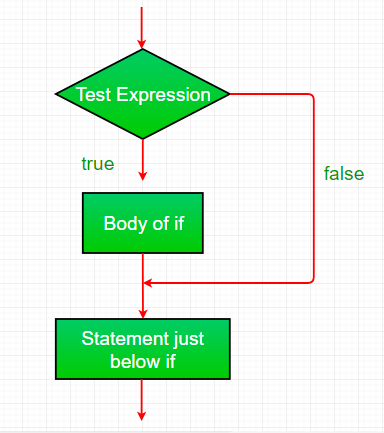Example:

 `// Java program to illustrate If statement``class` `IfDemo``{``    ``public` `static` `void` `main(String args[])``    ``{``        ``int` `i = ``10``;`` ` `        ``if` `(i > ``15``)``            ``System.out.println(``"10 is less than 15"``);`` ` `        ``// This statement will be executed``        ``// as if considers one statement by default``        ``System.out.println(``"I am Not in if"``);``    ``}``}`

Output:

```I am Not in if
```
• if-else: The if statement alone tells us that if a condition is true it will execute a block of statements and if the condition is false it won’t. But what if we want to do something else if the condition is false. Here comes the else statement. We can use the else statement with if statement to execute a block of code when the condition is false.
Syntax:
```if (condition)
{
// Executes this block if
// condition is true
}
else
{
// Executes this block if
// condition is false
}
```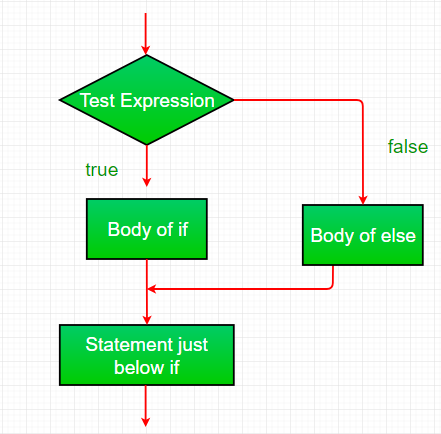Example:

 `// Java program to illustrate if-else statement``class` `IfElseDemo``{``    ``public` `static` `void` `main(String args[])``    ``{``        ``int` `i = ``10``;`` ` `        ``if` `(i < ``15``)``            ``System.out.println(``"i is smaller than 15"``);``        ``else``            ``System.out.println(``"i is greater than 15"``);``    ``}``}`

Output:

`i is smaller than 15`
• nested-if: A nested if is an if statement that is the target of another if or else. Nested if statements means an if statement inside an if statement. Yes, java allows us to nest if statements within if statements. i.e, we can place an if statement inside another if statement.
Syntax:
```if (condition1)
{
// Executes when condition1 is true
if (condition2)
{
// Executes when condition2 is true
}
}
```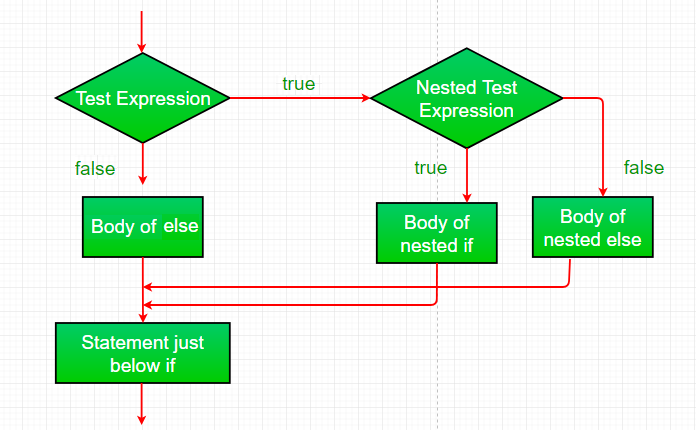Example:

 `// Java program to illustrate nested-if statement``class` `NestedIfDemo``{``    ``public` `static` `void` `main(String args[])``    ``{``        ``int` `i = ``10``;`` ` `        ``if` `(i == ``10``)``        ``{``            ``// First if statement``            ``if` `(i < ``15``)``                ``System.out.println(``"i is smaller than 15"``);`` ` `            ``// Nested - if statement``            ``// Will only be executed if statement above``            ``// it is true``            ``if` `(i < ``12``)``                ``System.out.println(``"i is smaller than 12 too"``);``            ``else``                ``System.out.println(``"i is greater than 15"``);``        ``}``    ``}``}`

Output:

```i is smaller than 15
i is smaller than 12 too
```
• if-else-if ladder: Here, a user can decide among multiple options.The if statements are executed from the top down. As soon as one of the conditions controlling the if is true, the statement associated with that if is executed, and the rest of the ladder is bypassed. If none of the conditions is true, then the final else statement will be executed.
```if (condition)
statement;
else if (condition)
statement;
.
.
else
statement;
```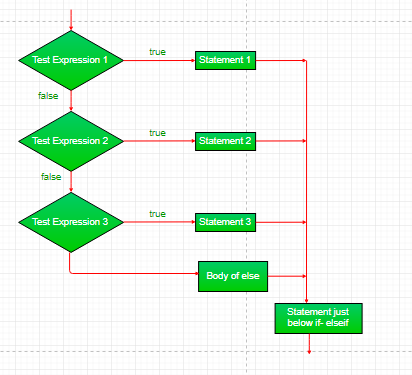Example:

 `// Java program to illustrate if-else-if ladder``class` `ifelseifDemo``{``    ``public` `static` `void` `main(String args[])``    ``{``        ``int` `i = ``20``;`` ` `        ``if` `(i == ``10``)``            ``System.out.println(``"i is 10"``);``        ``else` `if` `(i == ``15``)``            ``System.out.println(``"i is 15"``);``        ``else` `if` `(i == ``20``)``            ``System.out.println(``"i is 20"``);``        ``else``            ``System.out.println(``"i is not present"``);``    ``}``}`

Output:

```i is 20
```
• switch-case The switch statement is a multiway branch statement. It provides an easy way to dispatch execution to different parts of code based on the value of the expression.
Syntax:
```switch (expression)
{
case value1:
statement1;
break;
case value2:
statement2;
break;
.
.
case valueN:
statementN;
break;
default:
statementDefault;
}```
• Expression can be of type byte, short, int char or an enumeration. Beginning with JDK7, expression can also be of type String.
• Dulplicate case values are not allowed.
• The default statement is optional.
• The break statement is used inside the switch to terminate a statement sequence.
• The break statement is optional. If omitted, execution will continue on into the next case.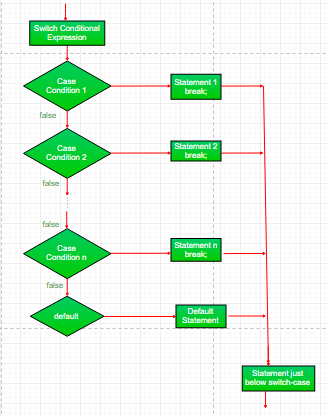Example:

 `// Java program to illustrate switch-case``class` `SwitchCaseDemo``{``    ``public` `static` `void` `main(String args[])``    ``{``        ``int` `i = ``9``;``        ``switch` `(i)``        ``{``        ``case` `0``:``            ``System.out.println(``"i is zero."``);``            ``break``;``        ``case` `1``:``            ``System.out.println(``"i is one."``);``            ``break``;``        ``case` `2``:``            ``System.out.println(``"i is two."``);``            ``break``;``        ``default``:``            ``System.out.println(``"i is greater than 2."``);``        ``}``    ``}``}`

Output:

```i is greater than 2.
```
• jump: Java supports three jump statement: break, continue and return. These three statements transfer control to other part of the program.
1. Break: In Java, break is majorly used for:
• Terminate a sequence in a switch statement (discussed above).
• To exit a loop.
• Used as a “civilized” form of goto.

Using break to exit a Loop

Using break, we can force immediate termination of a loop, bypassing the conditional expression and any remaining code in the body of the loop.
Note: Break, when used inside a set of nested loops, will only break out of the innermost loop.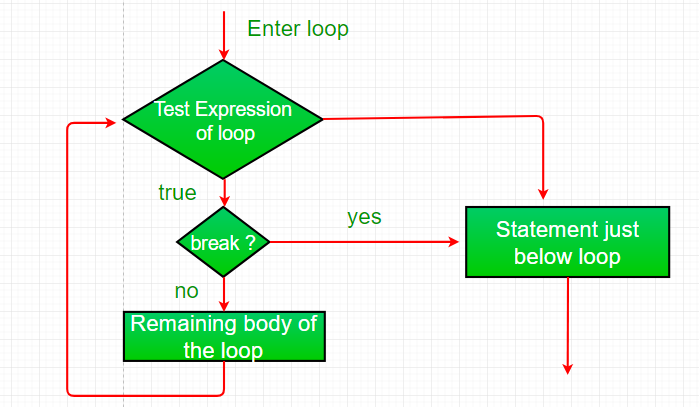Example:

 `// Java program to illustrate using``// break to exit a loop``class` `BreakLoopDemo``{``    ``public` `static` `void` `main(String args[])``    ``{``        ``// Initially loop is set to run from 0-9``        ``for` `(``int` `i = ``0``; i < ``10``; i++)``        ``{``            ``// terminate loop when i is 5.``            ``if` `(i == ``5``)``                ``break``;`` ` `            ``System.out.println(``"i: "` `+ i);``        ``}``        ``System.out.println(``"Loop complete."``);``    ``}``}`

Output:

```i: 0
i: 1
i: 2
i: 3
i: 4
Loop complete.
```

Using break as a Form of Goto

Java does not have a goto statement because it provides a way to branch in an arbitrary and unstructured manner. Java uses label. A Label is use to identifies a block of code.
Syntax:

```label:
{
statement1;
statement2;
statement3;
.
.
}```

Now, break statement can be use to jump out of target block.
Note: You cannot break to any label which is not defined for an enclosing block.
Syntax:

`break label;`

Example:

 `// Java program to illustrate using break with goto``class` `BreakLabelDemo``{``    ``public` `static` `void` `main(String args[])``    ``{``        ``boolean` `t = ``true``;`` ` `        ``// label first``        ``first:``        ``{``            ``// Illegal statement here as label second is not``            ``// introduced yet break second;``            ``second:``            ``{``                ``third:``                ``{``                    ``// Before break``                    ``System.out.println(``"Before the break statement"``);`` ` `                    ``// break will take the control out of``                    ``// second label``                    ``if` `(t)``                        ``break` `second;``                    ``System.out.println(``"This won't execute."``);``                ``}``                ``System.out.println(``"This won't execute."``);``            ``}`` ` `            ``// First block``            ``System.out.println(``"This is after second block."``);``        ``}``    ``}``}`

Output:

```Before the break.
This is after second block.
```
2. Continue: Sometimes it is useful to force an early iteration of a loop. That is, you might want to continue running the loop but stop processing the remainder of the code in its body for this particular iteration. This is, in effect, a goto just past the body of the loop, to the loop’s end. The continue statement performs such an action.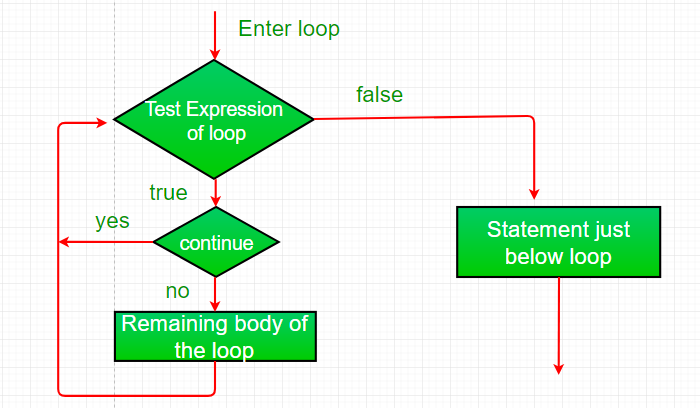Example:
 `// Java program to illustrate using``// continue in an if statement``class` `ContinueDemo``{``    ``public` `static` `void` `main(String args[])``    ``{``        ``for` `(``int` `i = ``0``; i < ``10``; i++)``        ``{``            ``// If the number is even``            ``// skip and continue``            ``if` `(i%``2` `== ``0``)``                ``continue``;`` ` `            ``// If number is odd, print it``            ``System.out.print(i + ``" "``);``        ``}``    ``}``}`

Output:

```1 3 5 7 9
```
3. Return:The return statement is used to explicitly return from a method. That is, it causes a program control to transfer back to the caller of the method.
Example:
 `// Java program to illustrate using return``class` `Return``{``    ``public` `static` `void` `main(String args[])``    ``{``        ``boolean` `t = ``true``;``        ``System.out.println(``"Before the return."``);``     ` `        ``if` `(t)``            ``return``;`` ` `        ``// Compiler will bypass every statement ``        ``// after return``        ``System.out.println(``"This won't execute."``);``    ``}``}`

Output:

```Before the return.
```

This article is contributed by Anuj Chauhan and Harsh Aggarwal. If you like GeeksforGeeks and would like to contribute, you can also write an article using contribute.geeksforgeeks.org or mail your article to contribute@geeksforgeeks.org. See your article appearing on the GeeksforGeeks main page and help other Geeks.